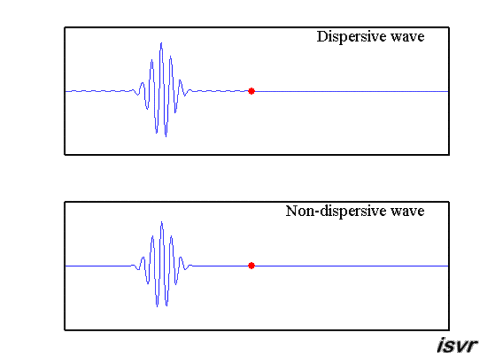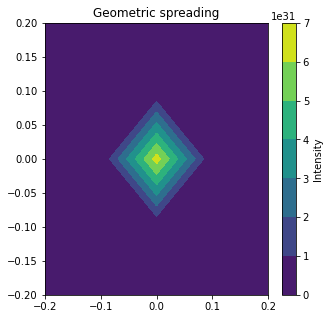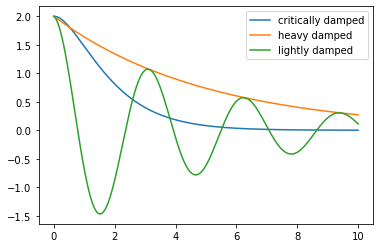# Attenuation#

Waves

In this notebook, the different types of attenuation will be discussed. Group velocity and dispersion are discussed here (link?).

Attenuation is the loss of intensity as waves travel through a medium.

import numpy as np
import matplotlib.pyplot as plt


## 1. Dispersion#

In dispersion, higher frequency waves travel faster than lower frequency waves. The energy spreads out thus leaving less intensity for the pulses.Energy spreads out as a function of area. Thus, intensity decreases away from the source. It is important to look at the dimensions of spreading, i.e. water waves pread in 2D so the area is $$2\pi r$$. Body waves spread out in 3D, so the area to consider is $$2 \pi r^2$$. Geometric spreading is defined as initial intensity divided by the area as a function of distance from the source so

$\frac{I_0}{area}$

For example, $$\frac{I_0}{2\pi r}$$ for water waves.

x = np.arange(-0.5,0.5,0.1)
y = np.arange(-0.5,0.5,0.1)
X, Y = np.meshgrid(x,y)

I_0 = 10
r = (X**2+Y**2)**0.5 # calculate radius
z = I_0/(2 * np.pi * r**2) # geometric spreading
fig, ax= plt.subplots(figsize = (5,5))
CS = plt.contourf(X,Y,z)
plt.xlim(-0.2, 0.2)
plt.ylim(-0.2, 0.2)
cbar = plt.colorbar()
cbar.set_label('Intensity', rotation=90)
plt.show()## 3. Scattering#

Scattering occurs when a medium’s impedance has length scales that are smaller than the wavelength of the wave. These changes can also cause reflection and transmission.## 4. Intrinsic attenuation#

Gradual loss of energy from the wave, due to materials not being perfectly elastic. This energy is converted into heat. Attenuation can cause damping of particle motion. If there is a friction that opposes the motion of oscillation, the equation of motion for the particle becomes

$m \frac{d^2 y}{d t^2} = -sy -r \frac{dy}{dt}$

This equation has three solutions:

• Heavy damping: strongest case of attenuation, prohibiting the particle to oscillate. Solution of the form $$y=Ce^{-at}$$. So damping in this situation is stronger than the restoring force.

• Critical damping: solution of the form $$y=(A+Bt)e^{-pt}$$. Less attenuation than in heavy damping case, but returns to rest position more quickly still if it has zero velocity at the start.

• Light damping is of the form $$y=y_0e^{-bt}\cos(\omega t + \phi)$$.

t = np.linspace(0,10,1001)
a = 2
w = 2
damping_factor = (1+1*t)*np.exp(-t)
y = damping_factor * a
plt.plot(t,y, label = 'critically damped')

damping_factor2 = np.exp(-0.2*x)
y2 = damping_factor2 * a
plt.plot(x,y2, label = 'heavy damped')

damping_factor3 = np.exp(-0.2*x)
y2 = damping_factor2 * a * np.cos(w * x)
plt.plot(x,y2, label = 'lightly damped')
# plt.legend()

plt.legend()
plt.show()## Quality factor#

The quality factor is of the form

$Q = \frac{m \omega_0}{r}$

where $$\omega_0 =\sqrt{\frac{s}{m}}$$, the natural frequency.